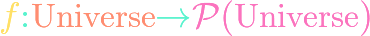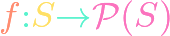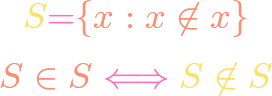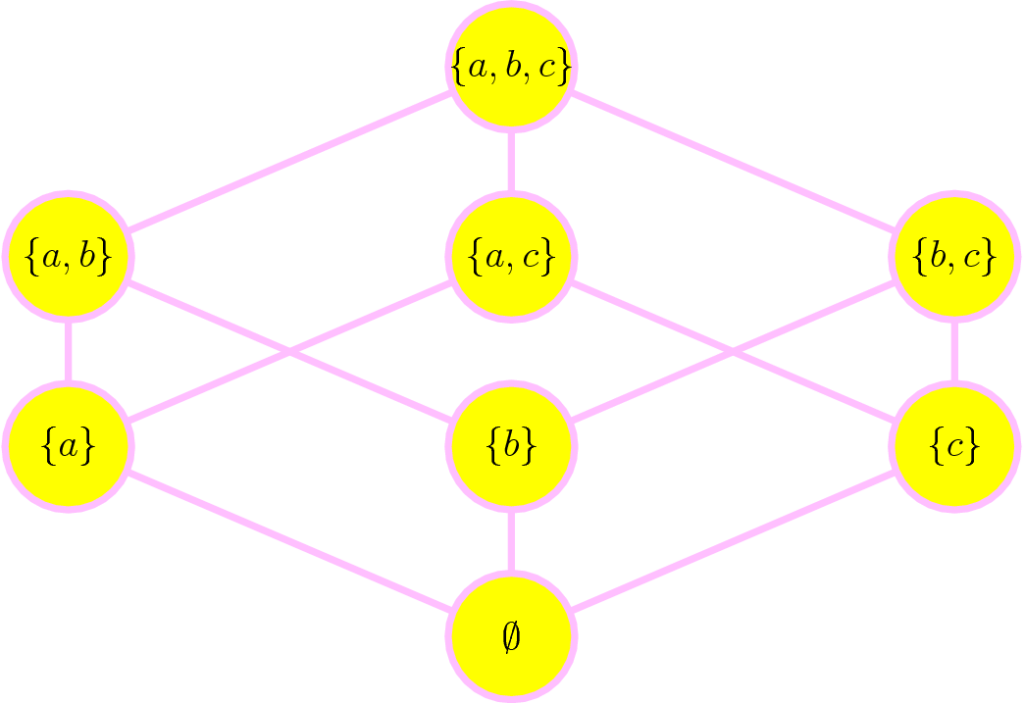# Foundations## Cantor’s Paradox in Set Theory

https://www.youtube.com/watch?v=oTXvwc3k8ag Cantor’s paradox derives a contradiction from the assumption that there is a set of all sets. Like Russell’s paradox, this proves that there can be no set whose elements are all sets; in other words, the mathematical universe is not a set. The main tool is Cantor’s theorem, a topic on which we have …## Cantor’s Theorem in Set Theory

https://www.youtube.com/watch?v=7FQu540d3-A By repeatedly taking the power set $mathcal{P}(S)$ of an infinite set $S$, Cantor’s theorem shows that these new infinities get strictly “bigger and bigger.” So there exists an infinite hierarchy of infinities in the sense that the lower infinities cannot map surjectively onto the higher infinities (but injective maps are possible).## Bertrand Russell’s Paradox in Set Theory

https://www.youtube.com/watch?v=Drsqa4XFgJQ Bertrand Russell’s paradox in set theory shook the foundations of the mathematical world in 1901 by showing that naive set theory leads to a contradiction. This caused mathematicians to adopt the convention that one cannot summon elements directly from the entire mathematical universe into a set, and that there is no set of all …## Subset Relation is a Partial Order

https://www.youtube.com/watch?v=cCEvHZgq188 One of the most powerful and general concepts in math is the idea of a partial order. We show that, given that we are working within a universl set, its subsets fall into a partial order under the subset relation $Xsubseteq Y$. This means that the subset relation is reflexive, antisymmetric, and transitive.